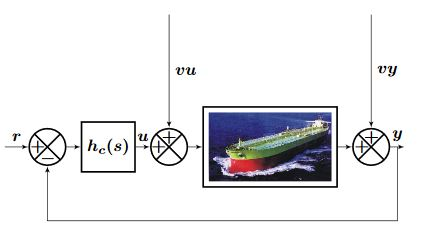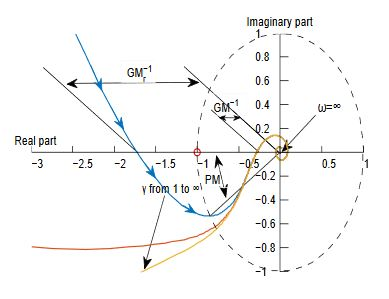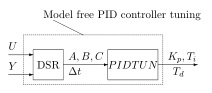## Main Topics:  Advanced Control Theory

1. Part1- System Theory: Models of systems. Continuous and discrete time domain models. State space models. Poles and zeroes in MIMO systems. Stability. Multivariable frequency analysis.

2. Part2- Continuous time LQ Optimal Control: The Maximum principle. Standard Linear Quadratic (LQ) optimal control. LQ optimal control with disturbances and integral action. Tracking control. The Algebraic Riccati Equation (ARE).

3. Part3- Discrete time LQ Optimal Control: Maximum Principle for discrete time systems. Discrete LQ optimal control. Disturbances and integral action. tracking control. The Discrete Algebraic Riccati Equation (DARE). Discrete LQ optimal control with integral action: A simple controller on incremental form for MIMO systems

4. Part4- Special: The Kalman Filter and LQ Optimal Control: LQG control.

5. Part 5- Tuning PID controllers directly from observed data. Model free PID controller tuning. Design of PID controllers for prescribed relative time delay error, GM etc.

Direct PID controller tuning: Model_free_tuning

References:
1. The figures above are from Di Ruscio and Dalen (2017)Updated 2019 david.di.ruscio@usn.no